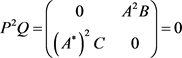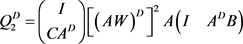﻿ Hamilton矩阵广义逆的表示

# Hamilton矩阵广义逆的表示The Representation of the Generalized Inverse of a Hamilton Matrix

Abstract: The aim of this paper is to establish an explicit representation of the Drazin inverse of a Hamilton matrixunder certain conditions. Then we give a formula for the Drazin inverse of a Hamilton matrixwhen the generalized Schur complement.

1. 引言

Hamilton体系是由19世纪爱尔兰数学家兼物理学家W. R. Hamilton从几何光学着手创建起来的理论模式，而后他将此模式创造性的应用于经典力学，得到了在经典力学范畴内的又一种力学描述形式—Hamilton力学 。作为Hamilton系统中最简单且最基本的形式，有限维线性Hamilton系统所对应的系数矩阵如下：

$H=\left(\begin{array}{cc}A& B\\ C& -{A}^{*}\end{array}\right)$ ,

${A}^{D}A{A}^{D}={A}^{D}$ , $A{A}^{D}={A}^{D}A$ , ${A}^{k+1}{A}^{D}={A}^{k}$ ,

Drazin逆在Markov链、奇异微分方程和差分方程、迭代方法等各领域起着重要作用 。本文主要研究了Hamilton矩阵在一定条件下的Drazin逆，并给出了一些 ${H}^{D}$ 的表达式。

2. 引理

${\left(P+Q\right)}^{D}=\underset{i=0}{\overset{s-1}{\sum }}{Q}^{i}{\left({P}^{D}\right)}^{i+1}$ .

${M}^{D}=\left(\begin{array}{c}I\\ C{A}^{D}\end{array}\right){\left[{\left(AW\right)}^{D}\right]}^{2}A\left(\begin{array}{cc}I& {A}^{D}B\end{array}\right)$ , $W=A{A}^{D}+{A}^{D}BC{A}^{D}$ .

3. 主要结论及证明

${H}^{D}=\left(\begin{array}{cc}{A}^{D}& B{\left({\left({A}^{\ast }\right)}^{D}\right)}^{2}-AB{\left({\left({A}^{\ast }\right)}^{D}\right)}^{3}\\ C{\left({A}^{D}\right)}^{2}-{A}^{\ast }C{\left({A}^{D}\right)}^{3}& -{\left({A}^{\ast }\right)}^{D}\end{array}\right)$ .

$H=\left(\begin{array}{cc}A& 0\\ 0& -{A}^{*}\end{array}\right)\text{+}\left(\begin{array}{cc}0& B\\ C& 0\end{array}\right)$ ,

$P=\left(\begin{array}{cc}A& 0\\ 0& -{A}^{*}\end{array}\right)$ , $Q=\left(\begin{array}{cc}0& B\\ C& 0\end{array}\right)$ ,,,

$\begin{array}{c}{H}^{D}={\left(P+Q\right)}^{D}={P}^{D}+Q{\left({P}^{D}\right)}^{2}+PQ{\left({P}^{D}\right)}^{3}\\ =\left(\begin{array}{cc}{A}^{D}& 0\\ 0& -{\left({A}^{\ast }\right)}^{D}\end{array}\right)+\left(\begin{array}{cc}0& B{\left({\left({A}^{\ast }\right)}^{D}\right)}^{2}\\ C{\left({A}^{D}\right)}^{2}& 0\end{array}\right)+\left(\begin{array}{cc}0& -AB{\left({\left({A}^{\ast }\right)}^{D}\right)}^{3}\\ -{A}^{\ast }C{\left({A}^{D}\right)}^{3}& 0\end{array}\right)\\ =\left(\begin{array}{cc}{A}^{D}& B{\left({\left({A}^{\ast }\right)}^{D}\right)}^{2}-AB{\left({\left({A}^{\ast }\right)}^{D}\right)}^{3}\\ C{\left({A}^{D}\right)}^{2}-{A}^{\ast }C{\left({A}^{D}\right)}^{3}& -{\left({A}^{\ast }\right)}^{D}\end{array}\right).\end{array}$

${H}^{D}=\left(\begin{array}{cc}A{\left(BC\right)}^{D}-B{A}^{*}{\left({\left(CB\right)}^{D}\right)}^{2}C& B{\left(CB\right)}^{D}\\ {\left(CB\right)}^{D}C& -{A}^{*}{\left(CB\right)}^{D}+CAB{\left({\left(CB\right)}^{D}\right)}^{2}\end{array}\right)$ .

$H=\left(\begin{array}{cc}0& B\\ C& 0\end{array}\right)\text{+}\left(\begin{array}{cc}A& 0\\ 0& -{A}^{*}\end{array}\right)$ ,

$P=\left(\begin{array}{cc}0& B\\ C& 0\end{array}\right)$ , $Q=\left(\begin{array}{cc}A& 0\\ 0& -{A}^{*}\end{array}\right)$ ,

${P}^{D}=\left(\begin{array}{cc}0& B{\left(CB\right)}^{D}\\ {\left(CB\right)}^{D}C& 0\end{array}\right)$ , ${P}^{\pi }=\left(\begin{array}{cc}{\left(BC\right)}^{\pi }& 0\\ 0& {\left(CB\right)}^{\pi }\end{array}\right)$ .

${P}^{2}Q=\left(\begin{array}{cc}BCA& 0\\ 0& -CB{A}^{*}\end{array}\right)=0$ ,

$Q{P}^{\pi }=\left(\begin{array}{cc}A{\left(BC\right)}^{\pi }& 0\\ 0& -{A}^{*}{\left(CB\right)}^{\pi }\end{array}\right)=0$ ,

$\begin{array}{c}{H}^{D}={\left(P+Q\right)}^{D}={P}^{D}+Q{\left({P}^{D}\right)}^{2}+PQ{\left({P}^{D}\right)}^{3}\\ =\left(\begin{array}{cc}0& B{\left(CB\right)}^{D}\\ {\left(CB\right)}^{D}C& 0\end{array}\right)+\left(\begin{array}{cc}A{\left(BC\right)}^{D}& 0\\ 0& -{A}^{*}{\left(CB\right)}^{D}\end{array}\right)+\left(\begin{array}{cc}-B{A}^{*}{\left({\left(CB\right)}^{D}\right)}^{2}C& 0\\ 0& CAB{\left({\left(CB\right)}^{D}\right)}^{2}\end{array}\right)\\ =\left(\begin{array}{cc}A{\left(BC\right)}^{D}-B{A}^{*}{\left({\left(CB\right)}^{D}\right)}^{2}C& B{\left(CB\right)}^{D}\\ {\left(CB\right)}^{D}C& -{A}^{*}{\left(CB\right)}^{D}+CAB{\left({\left(CB\right)}^{D}\right)}^{2}\end{array}\right).\end{array}$

${H}^{D}=\left(\begin{array}{cc}{A}^{D}+AB{\left({\left(CB\right)}^{D}\right)}^{2}C& B{\left(CB\right)}^{D}\\ {\left(CB\right)}^{D}C& -{\left({A}^{*}\right)}^{D}-{A}^{*}{\left(CB\right)}^{D}\end{array}\right)$ .

$H=\left(\begin{array}{cc}A& 0\\ 0& -{A}^{*}\end{array}\right)\text{+}\left(\begin{array}{cc}0& B\\ C& 0\end{array}\right)$ ,

$P=\left(\begin{array}{cc}A& 0\\ 0& -{A}^{*}\end{array}\right)$ , $Q=\left(\begin{array}{cc}0& B\\ C& 0\end{array}\right)$ ,

${P}^{2}Q=\left(\begin{array}{cc}0& {A}^{2}B\\ {\left({A}^{*}\right)}^{2}C& 0\end{array}\right)=0$ ,

$PQ+QP=\left(\begin{array}{cc}0& AB-B{A}^{*}\\ -{A}^{*}C+CA& 0\end{array}\right)=0$ ,

$\begin{array}{c}{H}^{D}={\left(P+Q\right)}^{D}={P}^{D}+\left(P+Q\right){\left({Q}^{D}\right)}^{2}\\ =\left(\begin{array}{cc}{A}^{D}& 0\\ 0& -{\left(A*\right)}^{D}\end{array}\right)+\left(\begin{array}{cc}A& B\\ C& -{A}^{*}\end{array}\right){\left(\begin{array}{cc}0& B{\left(CB\right)}^{D}\\ {\left(CB\right)}^{D}C& 0\end{array}\right)}^{2}\\ =\left(\begin{array}{cc}{A}^{D}+AB{\left({\left(CB\right)}^{D}\right)}^{2}C& B{\left(CB\right)}^{D}\\ {\left(CB\right)}^{D}C& -{\left({A}^{*}\right)}^{D}-{A}^{*}{\left(CB\right)}^{D}\end{array}\right).\end{array}$

${H}^{D}=\left(\begin{array}{cc}A& B\\ C& -{A}^{*}\end{array}\right){E}^{2}$ ,

${E}^{l}={F}^{l}+\underset{i=1}{\overset{k}{\sum }}{F}^{i+l}\left(\begin{array}{cc}{A}^{i}{A}^{\pi }& 0\\ C{A}^{i-1}{A}^{\pi }& 0\end{array}\right),l\ge 1$ ,

${F}^{l}={\left({Q}_{2}^{D}\right)}^{l}=\left(\begin{array}{c}I\\ C{A}^{D}\end{array}\right){\left[{\left(AW\right)}^{D}\right]}^{l+1}{A}^{2}{A}^{D}\left(\begin{array}{cc}I& {A}^{D}B\end{array}\right)$ ,

$W=A{A}^{D}+{A}^{D}BC{A}^{D}$ .

$H=\left(\begin{array}{cc}A& B\\ C& C{A}^{D}B\end{array}\right)=\left(\begin{array}{cc}0& {A}^{\pi }B\\ 0& 0\end{array}\right)+\left(\begin{array}{cc}A& A{A}^{D}B\\ C& C{A}^{D}B\end{array}\right)$ ,

$P=\left(\begin{array}{cc}0& {A}^{\pi }B\\ 0& 0\end{array}\right)$ , $Q=\left(\begin{array}{cc}A& A{A}^{D}B\\ C& C{A}^{D}B\end{array}\right)$ ,

${H}^{D}=\left(P+Q\right){\left({Q}^{D}\right)}^{2}$ , (1)

${Q}_{1}=\left(\begin{array}{cc}A{A}^{\pi }& 0\\ C{A}^{\pi }& 0\end{array}\right)$ , ${Q}_{2}=\left(\begin{array}{cc}{A}^{2}{A}^{D}& A{A}^{D}B\\ CA{A}^{D}& C{A}^{D}B\end{array}\right)$ ,

${Q}^{D}=\underset{i=0}{\overset{k}{\sum }}{\left({Q}_{2}^{D}\right)}^{i+1}{Q}_{1}^{i}$ ,, $W=A{A}^{D}+{A}^{D}BC{A}^{D}$ ,

${\left({Q}_{2}^{D}\right)}^{i}=\left(\begin{array}{c}I\\ C{A}^{D}\end{array}\right){\left[{\left(AW\right)}^{D}\right]}^{i+1}{A}^{2}{A}^{D}\left(\begin{array}{cc}I& {A}^{D}B\end{array}\right)$ , $W=A{A}^{D}+{A}^{D}BC{A}^{D}$ ,

$E={Q}^{D}$ , $F={Q}_{2}^{D}$ ，则

${E}^{l}={F}^{l}+\underset{i=1}{\overset{k}{\sum }}{F}^{i+l}\left(\begin{array}{cc}{A}^{i}{A}^{\pi }& 0\\ C{A}^{i-1}{A}^{\pi }& 0\end{array}\right),l\ge 1$ ,

$E={Q}^{D}$ 代入(1)，证毕。

NOTES

*通讯作者。

 冯康. 哈密尔顿系统的辛几何算法[M]. 杭州: 浙江科技出版社, 2003.

 吴德玉, 阿拉坦仓. 分块算子矩阵谱理论及其应用[M]. 北京: 科学出版社, 2013.

 邢立刚. 一类无穷维Hamilton算子谱的刻画[M]. 呼和浩特: 内蒙古大学出版社, 2007.

 Drzain, M.P. (1958) Pseudo-Inverses in Associative Rings and Semigroups. The American Mathematical Monthly, 65, 506-514.
https://doi.org/10.1080/00029890.1958.11991949

 Bru, R., Climent, J.J. and Neumann, M. (1995) On the Index of Block Upper Triangular Matrices. SIAM Journal on Matrix Analysis and Applications, 16, 436-447.
https://doi.org/10.1137/S0895479892235587

 Xia, L. and Deng, B. (2017) The Drazin Inverse of the Sum of Two Matrices and Its Applications. Filomat, 31, 5151-5158.
https://doi.org/10.2298/FIL1716151X

 Catral, M., Olesky, D.D. and Van Den Driessche, P. (2009) Block Representations of the Drazin Inverse of a Bipartite Matrix. The Electronic Journal of Linear Algebra, 18, 98-107.
https://doi.org/10.13001/1081-3810.1297

 Hartwig, R.E., Wang, G. and Wei, Y.M. (2001) Some Additive Results on Drazin Inverse. Linear Algebra and Its Applications, 322, 207-217.
https://doi.org/10.1016/S0024-3795(00)00257-3

 Miao, J. (1989) Results of Drazin Inverse of a 2 × 2 Block Matrices. Journal of Shanghai Normal University, 18, 25-31.

Top